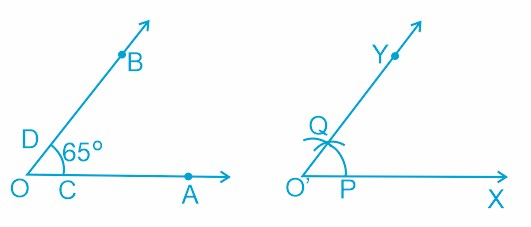#### Draw an angle of 65o and draw an angle equal to this angle, using ruler and compasses.Steps of construction:
(i) Draw an angle  using the protractor.
(ii) Now draw another line O'X.
(iii) Take O as a centre and any convenient radius, draw an arc cutting OA at C and OB at D.
(iv) Take O' as center and the same radius as previously drawn, draw an arc cutting O'X at P.
(v) Take radius equal to CD and P as centre, draw the arc passing through Q.
(vi) Now Join O'Q and produce it to Y.